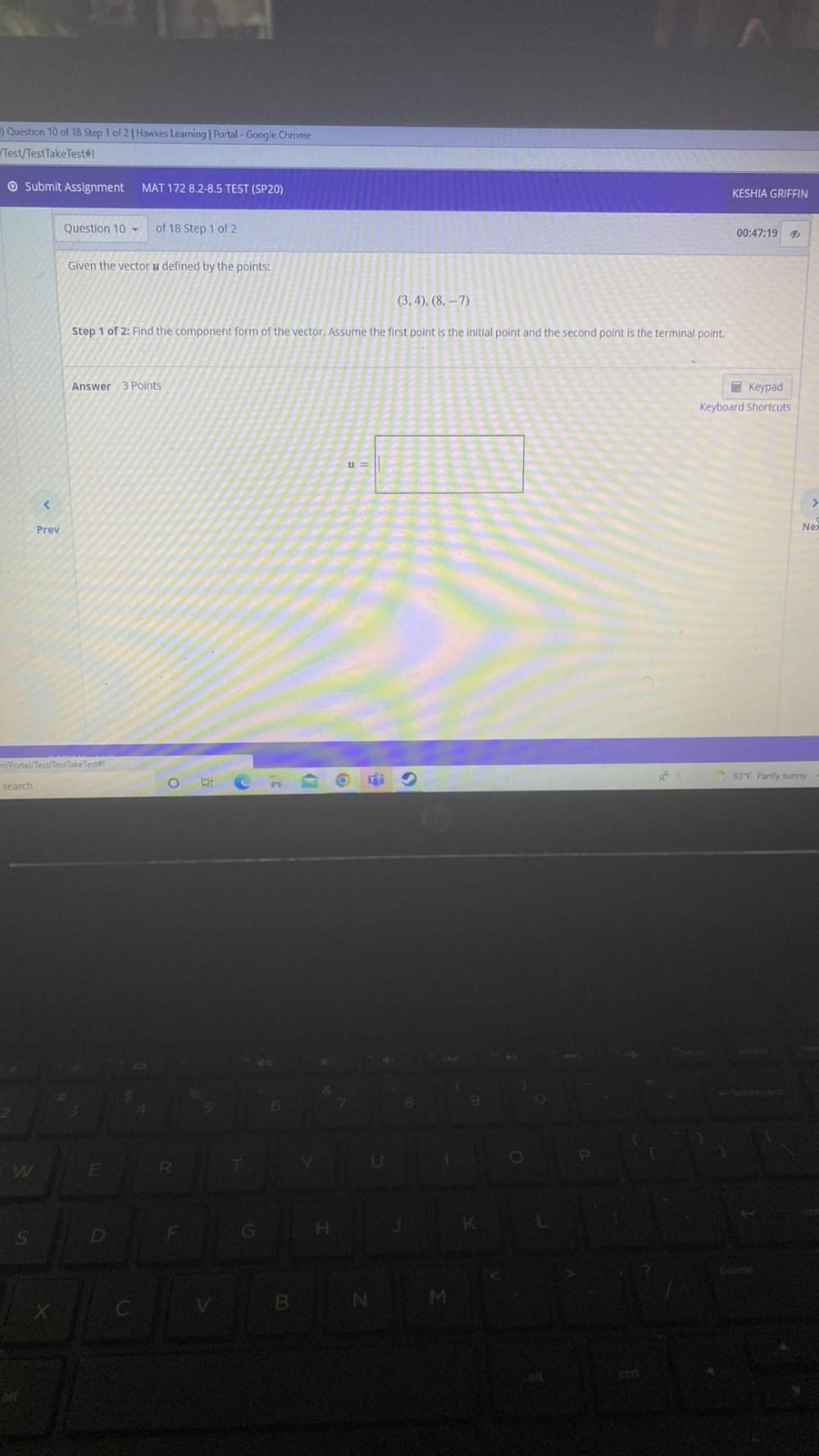Question:

# Given the vector u defined by the points: (3,4), (8, -7). Find the component form of the vector. Assume the first point is the initial point and the second point is the terminal point.Given the vector u defined by the points: (3,4), (8, -7). Find the component form of the vector. Assume the first point is the initial point and the second point is the terminal point.